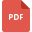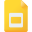# geometry tutorialsThe Implementation of Vedic Mathematics to Algebra and Geometry
Vedic Mathematics, Class 10, India, Algebra, GeometryGeometry: Understanding Parallel Lines
Mathematics, Grade 8, United States, Lines and AnglesGeometric Constructions
Mathematics, Grade 7, United States, GeometryBasics of Geometry ( POINTS, LINES, PLANES! )
Geometry, High School, United States, MathematicsGeometric Nets: For 3-D Shapes
Geometry, Grade 4, United States, MathematicsMEASUREMENT
Measurement, High School, GeometryWorksheet on Area of Rectangles
Geometry, Grade 3, United States, MathematicsDeductive Geometry- Geometry Using Triangle Congruence and Similarity
Geometry, High School, Congruence and SimilarityRectangles: Area and Perimeter Worksheet
Area, Grade 4, United States, Perimeter, Geometry, MathematicsIdentifying Angles: Acute Angle, Right Angle and Obtuse Angle
Right Triangle, Grade 5, United States, Lines and Angles, Mathematics, GeometryDescribing Geometric Solids
Solid Shapes, Grade 4, United States, GeometryFinding Area of Rectangles and Parallelogram
Parallelogram, Grade 6, United States, Mathematics, Area, GeometryGeometry, Grade 8, India, Mathematical Analysis, Mathematical EconomicsGeometric Shapes
Geometry, High School, United States, MathematicsBrief History of Geometry
Vedic Mathematics, Class 9, India, GeometryFinding the Area of a Triangle and Other Composite Shapes
Area, Grade 6, United States, Triangle, Geometry, MathematicsComplete Geometrical Transformations
Geometry, Grade 8, United States, MathematicsThe Sutras of Vedic Mathematics in Geometry
Vedic Mathematics, Class 9, IndiaGeometry: Perimeter of 2-D shapes
Geometry, Grade 3, United States, Perimeter